Reach Us+44 7456 035580
All submissions of the EM system will be redirected to Online Manuscript Submission System. Authors are requested to submit articles directly to Online Manuscript Submission System of respective journal.

# Investigating the Choline Chloride: Urea and Choline Chloride: Glycerol Deep Eutectic Solvents as Nanofluids Dispersants for Convective Heat Transfer

Ghassan H. Abdullah1, Noor Albayati2 and Mohammed Kadhom3*

1Department of Chemical Engineering, University of Tikrit, Saladin, Iraq

2Department of Chemical Engineering, Al-Muthana University, Al-Muthana, Iraq

3Department of Pathology, Al-Dour Technical Institute, Saladin, Iraq

*Corresponding Author:
Department of Pathology, Al- Dour Technical Institute, Saladin, Iraq
E-mail:
makbq6@mail.missouri.edu

Received date: 24/09/2018; Accepyed date: 22/11/2018; Published date: 29/11/2018

Visit for more related articles at Research & Reviews: Journal of Engineering and Technology

## Abstract

In this paper, two Deep Eutectic Solvents (DESs), choline chloride: urea and choline chloride: glycerol with molar ratios of 1:2, respectively, were investigated as dispersants for Al2O3 nanoparticles (NPs) to study the heat exchange. Because of the DESs’ high thermal properties, especially at high temperatures, they became good candidates for heat transfer applications. We prepared the DESs and examined their thermal conductivity as a new approach data using a thermal conductivity meter. Nanoparticles loading ratios of 1, 2, 3, 4, and 5 vol.% was proposed to inspect the thermal effect. Viscosity, friction factor (f), Nusselt number (Nu), Prandtl number (Pr), Reynolds number (Re), and heat transfer coefficient (h) relationships were studied in details under laminar flow conditions. Results showed that both of the pristine DESs had higher heat transfer coefficients than the nanofluids at all loadings, which attributed to the high heat capacity of the DESs than the NPs materials. Here, the heat transfer coefficient of the nanofluids decreased by increasing the NPs loading.

#### Keywords

Deep eutectic solvents, Nanofluid, Friction factor, Thermal conductivity, Nusselt number, heat transfer coefficient

#### Introduction

Nanofluids are the products of dispersing nanoparticles ( ≤ 100 nm) in fluids to upgrade their heat transfer properties . This type of fluids was gaining attention since it was introduced, more than 20 years ago , due to the easiness in preparation and remarkable improvement in thermal properties . Nanofluids were employed in a wide range of applications, including transformer oil , electronics industry [5-7], refrigerators and chillers [8,9], diesel combustion and electrical generators [10,11], pipes and heat exchangers [12,13], solar energy, nuclear reactors, space, cooling of machines, catalyst, and mass transfer .

Nanofluids are based on Brownian motion phenomenon, in which the particles are suspended in a fluid medium and the gravity has no effect due to their small size . Different nanoparticles (NPs) such as, silica , aluminum oxide , and copper  were added to water , ethylene glycol , glycol , etc. to synthesize nanofluids mixtures. Many researchers assumed the nanofluids as a single-phase liquid, which neglected many physical impacts and calculations. Therefore, challenges to describe the suspension results conflicting, and poor mechanism understanding was reported .

“Deep eutectic solvents” is a term appeared in 2003  to describe solvents formed by the hydrogen bond interaction between two, or more , materials containing a hydrogen bond donor (HBD) and hydrogen bond acceptor (HBA) ; the final product possesses a lower melting point than its raw materials. The DESs often have melting points lower than 100°C, including a group of liquids at room temperature [23,24]. For example, the DES produced by mixing choline chloride (mp=302°C) and urea (mp=133°C) 1:2 molar ratio, respectively, has a melting point of 12°C . The DESs have many advantages, including, but not limited to, low flammability, low vapor pressure, inexpensive raw materials, environmentally green, broad electrical and thermal windows, biodegradable, safe, and easy to synthesize [25,26]. These exceptional properties made them good candidates for many applications .

Different DESs were reported as dispersants for nanoparticles which were used for different purposes. Martis et al. reported the use of choline chloride: urea DES to disperse pristine and oxidized multiwall carbon nanotubes, p-MWCNTs and o- MWCNTs, respectively. It was found that p-MWCNTs had low dispersion in the DES, while o-MWCNTs well dispersed. Abbott et al.  successfully dispersed SiC and Al2O3 nanoparticles in choline chloride: ethylene glycol DES, which used for silver electrodeposition. Mota-Morales et al.  injected MWCNTs in choline chloride: acrylic acid DES, which was prepared in different molar ratios, to manufacture macroporous poly (acrylic acid)-CNxMWCNT complexes. Recently, Fang et al.  have investigated the dispersion of graphene oxide nanoparticles in several DESs, which were introduced as nanofluids. Promising results were observed regarding dispersion, stability, and thermal conductivity. Nevertheless, the use of deep eutectic solvents as nanofluids media is still not common; in fact, much work is needed to utilize this kind of solvents .

Choline chloride: urea and choline chloride: glycerol deep eutectic solvents were intensively investigated since their raw materials are safe and common [20,32,33]. These DESs have a wide thermal range , which promoted them for heat transfer applications. In this paper, the thermal conductivity of choline chloride: glycerol and choline chloride: urea DESs were measured and used to study the DESs as nanofluids dispersants for Al2O3 nanoparticles to examine the heat transfer. Friction factor, Nusselt number, Reynolds number, and heat transfer coefficient values were reported in the laminar flow region, due to DESs’ high viscosity (Table 1). The suggestion of using DESs for this type of application was due to their negligible vapor pressure, which made their usages safe, reliable, and overcame the water operation problems.

Table 1 : Greek symbols used during the study.

Nomenclature   Greek symbols
Cp specific heat capacity, J/kg K υ kinematic viscosity, m2/s
D tube diameter, m volume concentration, %
f friction factor ρ density, kg/m3
h heat transfer coefficient, W/m2 K α thermal diffusivity, m2/s
K thermal conductivity, W/m K μ viscosity, kg/ms
V mean velocity m/s Subscripts
Nu Nusselt number nf nanofluid
Re Reynolds number f base fluid
Pr Prandtl number

Materials

Choline chloride (ChCl, 99%, ACROS Organics), glycerol ( ≥ 99.5), and urea (99.0%) were purchased from Fisher Scientific (Pittsburgh, PA, USA) to prepare the DESs. A Millipore DI water system (18.2 MΩ.cm) produced by Synergy 185, EMD Millipore Corp. (Billerica, MA, USA) was used to provide water for cleaning purposes.

DESs Perpetration And Characterization

The DESs were prepared in accordance with a previously reported work . The chemicals were used as received without further treatments. Both choline chloride: urea (DES1) and choline chloride: glycerol (DES2) mixtures were synthesized in ratios of 1:2, respectively. The mixtures were prepared by mixing the reactants and heating them to 80°C with stirring for several hours. Ultimately, colorless products formed, which were stored in sealed containers to prevent any possible moisture.

The thermal conductivity test was conducted using a TLS-100 thermal conductivity meter (ThermTest Inc, Fredericton, NB, Canada). The results were obtained at room temperature and repeated three times to calculate the average; the standard deviation was calculated and reported as error bars.

System Set up

The suggested system to use in this work was designed as shown in Figure 1 and used to investigate the heat transfer from a hot fluid to the nanofluid. The process consisted of two loops, each has a tank, pump, flow meter, and two thermocouples; both loops are connected to a shell and tube heat exchanger. The suggested length of the heat exchanger was 1 m, involved 40 tubes of 5 mm diameter. Different flow rates, from 100 to 300 L/min, and NPs loading, from 1% to 5 %, were used for calculations. Viscosity and density values used in this study calculations were based on our previous report [32,33], while the heat capacity was employed as stated in a previous work . The mentioned properties were calculated for the nanofluids as well illustrated in the next section.

Data Processing

2-4-1 Density

The nanofluid density correlation was proposed by Pak and Cho , which can be defined as: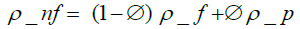(1)

where ρnf is the nanofluid density, ∅ the volume concentration of Al2O3 particles, ρf and ρp the base fluid and nanoparticles densities, respectively.

2-4-2 Specific heat capacity

Xuan and Roetzel  suggested a relation to calculate the nanofluid specific heat capacity as follows: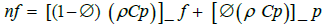(2)

where Cpnf, Cpf, and Cpp are the nanofluid, base fluid, and nanoparticles heat capacities, respectively.

2-4-3 Thermal conductivity

The nanofluid thermal conductivity was calculated using the equation introduced by Yu and Choi  as: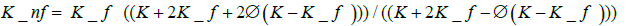(3)

where Knf, Kf, and K are the nanofluid, base fluid, and nanoparticles thermal conductivities, respectively.

2-4-4 Viscosity

Nanofluids viscosity was calculated using Einstein′s equation as suggested by Drew and Passman , which is applicable for spherical particles with fraction volume less than 5 vol%, as shown below: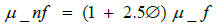(4)

where μnf and μf are the nanofluid and base fluid (DES) viscosities, respectively.

2-4-5 Friction factor

The friction factor for the pure DESs and nanofluids was estimated  as:

f = 64 / Re (5)

Reynolds number  can be calculated as below:

Re =VD /υ (6)

where V is the fluid velocity, D the tube diameter, and υ the Kinematic viscosity, which can be calculated by the following equation:

υ = μ / ρ (7)

2-4-6 Nusselt number

The base DESs and nanofluids Nusselt number was calculated by Seider-Tate equation  as presented below: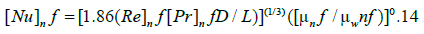(8)

where Nu is Nusselt number, Re Reynolds number, and Pr Prandtl number, which was calculated as :

Pr = ([Cp]_ nf μ _ nf ) / K _ nf (9)

It is worth noting that μnf and μwnf were assumed equal in this study, which neglects the second term of the above equation.

The heat transfer coefficient for forced convection in laminar flow range  can be calculated by:

Nu _ nf = hD / K _ nf

where h, D, and K are heat transfer coefficient, tube diameter, and thermal conductivity, respectively.

#### Results and Discussion

Effect of Temperature on Nanofluids Viscosities

The viscosity of the nanofluids was estimated using Einstein’s formula as modified by Drew and Passman for the two-phase mixture (equation 4). It is well known that the dynamic viscosity decreases by temperature increase due to the higher mobility of the ions, based on the Arrhenius equation . The viscosity of nanofluids was studied as a function of temperature within the range of 30°C to 70°C as presented in Figure 2a and 2b. From the figure, it can be observed that the NPs loading slightly affected the viscosity at low temperature. However, this influence diminished by increasing the temperature. On the other hand, the fluids followed Arrhenius behavior at all NPs loadings. Rising the temperature reduced the internal resistance of the molecules, which resulted in easy movement of the flow. Arrhenius equation is considered as a simple expression to describe this behavior, as long as the dependence of temperature on dynamic viscosity is concerned. The data showed identical curves for both DESs at different NPs ratios; however, DES1 showed higher viscosities than DES2. Urea has a higher effect on viscosity than glycerol due to its amide type, which could lead to forming self-hydrogen bonds between the chloride ions and urea .

Figure 2a: Viscosity of nanofluid as a function of nanoparticles volume and temperature for DES1.

Figure 2b: Viscosity of nanofluid as a function of nanoparticles volume and temperature for DES2.

The friction factor of pure DESs and nanofluids was calculated by equation 6 and its relationship with Reynold number was presented in Figure 3a and 3b for (a) DES1 and (b) DES2. Results showed that the base DES1 had a higher friction factor (2.662) than DES2 (1.382) at the same velocity (2.12 m/s) due to the higher viscosity of DES1, which attributed to the presence of urea . It can be noted, from both figures, that the friction factor decreased by increasing Re number at all NPs ratios. On the other hand, a tiny increase was observed by increasing the NPs ratios, which could be attributed to the high base fluid viscosity (no difference can be observed from the figure). It was reported that the nanoparticles increased the fluid’s viscosity, resulting in a higher friction factor due to the decrease in Re number [45,46]. However, in our DESs system, the friction factor was not affected by increasing the NPs load due to the high viscosity of the DESs. To evaluate our findings, the friction factor of DES1 and DES2 was compared to water at the loading ratio of 2%, with the consideration of different applied flow rates . The friction factor in this study was much higher, which could improve the heat transfer exchange . However, increasing the nanofluids viscosity could agglomerate the nanoparticles, leading to increasing the pumping system power.

Nusselt Number Relationship With Re and Pr Numbers

Nu number can be defined as the ratio of the convective to conductive heat transfer , which was calculated by equation 8. Figure 4a and 4b present Nu number as a function of Re number for the DESs at different NPs loadings. The results indicated that the base fluid has a higher Nu number than the nanofluids; Nu number decreased by increasing the NPs ratios but increased with Re number increasing . This is due to the effect of NPs on the density and viscosity of the nanofluid, by which Re number was estimated. Here, as Re number increased by decreasing the viscosity, DES2 had a higher Nu number than DES1. Figure 5a and 5b show the relationship of Nu number with Pr number. Pr number was calculated via Equation 9, where the thermal conductivity was practically measured in this work. The DES1 thermal conductivity was found to be 0.354 ± 0.06, while its value was 0.378 ± 0.07 for DES2. From the figure, DES2 showed higher Nu number than DES1 at the same Pr number. Also, Nu number decreased by NPs loading and Pr number increasing. The maximum Nusselt number was 57.116 for DES1 and 67.442 for DES2 at NPs loading ratio of 0%. Figure 6 illustrates the effect of NPs ratio on Pr number, where DES1 had higher values than DES2 due to its higher viscosity and lower thermal conductivity. By increasing the NPs ratio, Pr number decreased because of the decreasing in the heat capacity and increase in thermal conductivity. It was found that there are many factors might affect the heat transfer process, such as the friction force between the fluid and nanoparticles, Brownian force, agglomeration, and gravity .

Figure 5a: Nusselt number vs Prandtl number as a function of nanoparticles volume for DES1.

Figure 5b: Nusselt number vs Prandtl number as a function of nanoparticles volume for DES2.